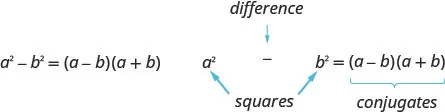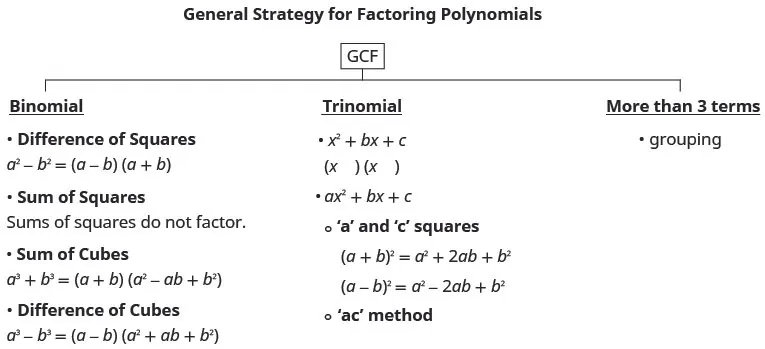Intermediate Algebra

# Key Concepts

Intermediate AlgebraKey Concepts

### Key Concepts

#### 6.1Greatest Common Factor and Factor by Grouping

• How to find the greatest common factor (GCF) of two expressions.
1. Step 1. Factor each coefficient into primes. Write all variables with exponents in expanded form.
2. Step 2. List all factors—matching common factors in a column. In each column, circle the common factors.
3. Step 3. Bring down the common factors that all expressions share.
4. Step 4. Multiply the factors.
• Distributive Property: If a, b, and c are real numbers, then
$a(b+c)=ab+acandab+ac=a(b+c)a(b+c)=ab+acandab+ac=a(b+c)$

The form on the left is used to multiply. The form on the right is used to factor.
• How to factor the greatest common factor from a polynomial.
1. Step 1. Find the GCF of all the terms of the polynomial.
2. Step 2. Rewrite each term as a product using the GCF.
3. Step 3. Use the “reverse” Distributive Property to factor the expression.
4. Step 4. Check by multiplying the factors.
• Factor as a Noun and a Verb: We use “factor” as both a noun and a verb.
$Noun:7 is afactorof 14Verb:factor3 from3a+3Noun:7 is afactorof 14Verb:factor3 from3a+3$
• How to factor by grouping.
1. Step 1. Group terms with common factors.
2. Step 2. Factor out the common factor in each group.
3. Step 3. Factor the common factor from the expression.
4. Step 4. Check by multiplying the factors.

#### 6.2Factor Trinomials

• How to factor trinomials of the form $x2+bx+c.x2+bx+c.$
1. Step 1. Write the factors as two binomials with first terms x. $x2+bx+c(x)(x)x2+bx+c(x)(x)$
2. Step 2. Find two numbers m and n that
$multiply toc,m·n=cadd tob,m+n=bmultiply toc,m·n=cadd tob,m+n=b$
3. Step 3. Use m and n as the last terms of the factors. $(x+m)(x+n)(x+m)(x+n)$
4. Step 4. Check by multiplying the factors.
• Strategy for Factoring Trinomials of the Form $x2+bx+cx2+bx+c$: When we factor a trinomial, we look at the signs of its terms first to determine the signs of the binomial factors.
$x2+bx+c(x+m)(x+n)Whencis positive,mandnhave the same sign.bpositivebnegativem,npositivem,nnegativex2+5x+6x2−6x+8(x+2)(x+3)(x−4)(x−2)same signssame signsWhencis negative,mandnhave opposite signs.x2+x−12x2−2x−15(x+4)(x−3)(x−5)(x+3)opposite signsopposite signsx2+bx+c(x+m)(x+n)Whencis positive,mandnhave the same sign.bpositivebnegativem,npositivem,nnegativex2+5x+6x2−6x+8(x+2)(x+3)(x−4)(x−2)same signssame signsWhencis negative,mandnhave opposite signs.x2+x−12x2−2x−15(x+4)(x−3)(x−5)(x+3)opposite signsopposite signs$
Notice that, in the case when m and n have opposite signs, the sign of the one with the larger absolute value matches the sign of b.
• How to factor trinomials of the form $ax2+bx+cax2+bx+c$ using trial and error.
1. Step 1. Write the trinomial in descending order of degrees as needed.
2. Step 2. Factor any GCF.
3. Step 3. Find all the factor pairs of the first term.
4. Step 4. Find all the factor pairs of the third term.
5. Step 5. Test all the possible combinations of the factors until the correct product is found.
6. Step 6. Check by multiplying.
• How to factor trinomials of the form $ax2+bx+cax2+bx+c$ using the “ac” method.
1. Step 1. Factor any GCF.
2. Step 2. Find the product ac.
3. Step 3. Find two numbers m and n that:
$Multiply toac.m·n=a·cAdd tob.m+n=bax2+bx+cMultiply toac.m·n=a·cAdd tob.m+n=bax2+bx+c$
4. Step 4. Split the middle term using m and n. $ax2+mx+nx+cax2+mx+nx+c$
5. Step 5. Factor by grouping.
6. Step 6. Check by multiplying the factors.

#### 6.3Factor Special Products

• Perfect Square Trinomials Pattern: If a and b are real numbers,
$a2+2ab+b2=(a+b)2a2−2ab+b2=(a−b)2a2+2ab+b2=(a+b)2a2−2ab+b2=(a−b)2$
• How to factor perfect square trinomials.
$Step 1.Does the trinomial fit the pattern?a2+2ab+b2a2−2ab+b2 Is the first term a perfect square?(a)2(a)2 Write it as a square. Is the last term a perfect square?(a)2(b)2(a)2(b)2 Write it as a square. Check the middle term. Is it2ab?(a)2↘2·a·b↙(b)2(a)2↘2·a·b↙(b)2 Step 2.Write the square of the binomial.(a+b)2(a−b)2 Step 3.Check by multiplying.Step 1.Does the trinomial fit the pattern?a2+2ab+b2a2−2ab+b2 Is the first term a perfect square?(a)2(a)2 Write it as a square. Is the last term a perfect square?(a)2(b)2(a)2(b)2 Write it as a square. Check the middle term. Is it2ab?(a)2↘2·a·b↙(b)2(a)2↘2·a·b↙(b)2 Step 2.Write the square of the binomial.(a+b)2(a−b)2 Step 3.Check by multiplying.$
• Difference of Squares Pattern: If $a,ba,b$ are real numbers,• How to factor differences of squares.
$Step 1.Does the binomial fit the pattern?a2−b2Is this a difference?____−____Are the first and last terms perfect squares?Step 2.Write them as squares.(a)2−(b)2Step 3.Write the product of conjugates.(a−b)(a+b)Step 4.Check by multiplying.Step 1.Does the binomial fit the pattern?a2−b2Is this a difference?____−____Are the first and last terms perfect squares?Step 2.Write them as squares.(a)2−(b)2Step 3.Write the product of conjugates.(a−b)(a+b)Step 4.Check by multiplying.$
• Sum and Difference of Cubes Pattern
$a3+b3=(a+b)(a2−ab+b2)a3−b3=(a−b)(a2+ab+b2)a3+b3=(a+b)(a2−ab+b2)a3−b3=(a−b)(a2+ab+b2)$
• How to factor the sum or difference of cubes.
1. Step 1. Does the binomial fit the sum or difference of cubes pattern?
Is it a sum or difference?
Are the first and last terms perfect cubes?
2. Step 2. Write them as cubes.
3. Step 3. Use either the sum or difference of cubes pattern.
4. Step 4. Simplify inside the parentheses
5. Step 5. Check by multiplying the factors.

#### 6.4General Strategy for Factoring Polynomials• How to use a general strategy for factoring polynomials.
1. Step 1. Is there a greatest common factor?
Factor it out.
2. Step 2. Is the polynomial a binomial, trinomial, or are there more than three terms?
If it is a binomial:
Is it a sum?
Of squares? Sums of squares do not factor.
Of cubes? Use the sum of cubes pattern.
Is it a difference?
Of squares? Factor as the product of conjugates.
Of cubes? Use the difference of cubes pattern.
If it is a trinomial:
Is it of the form $x2+bx+c?x2+bx+c?$ Undo FOIL.
Is it of the form $ax2+bx+c?ax2+bx+c?$
If a and c are squares, check if it fits the trinomial square pattern.
Use the trial and error or “ac” method.
If it has more than three terms:
Use the grouping method.
3. Step 3. Check.
Is it factored completely?
Do the factors multiply back to the original polynomial?

#### 6.5Polynomial Equations

• Polynomial Equation: A polynomial equation is an equation that contains a polynomial expression. The degree of the polynomial equation is the degree of the polynomial.
• Quadratic Equation: An equation of the form $ax2+bx+c=0ax2+bx+c=0$ is called a quadratic equation.
$a,b,care real numbers anda≠0a,b,care real numbers anda≠0$
• Zero Product Property: If $a·b=0,a·b=0,$ then either $a=0a=0$ or $b=0b=0$ or both.
• How to use the Zero Product Property
1. Step 1. Set each factor equal to zero.
2. Step 2. Solve the linear equations.
3. Step 3. Check.
• How to solve a quadratic equation by factoring.
1. Step 1. Write the quadratic equation in standard form, $ax2+bx+c=0.ax2+bx+c=0.$
2. Step 2. Factor the quadratic expression.
3. Step 3. Use the Zero Product Property.
4. Step 4. Solve the linear equations.
5. Step 5. Check. Substitute each solution separately into the original equation.
• Zero of a Function: For any function f, if $f(x)=0,f(x)=0,$ then x is a zero of the function.
• How to use a problem solving strategy to solve word problems.
1. Step 1. Read the problem. Make sure all the words and ideas are understood.
2. Step 2. Identify what we are looking for.
3. Step 3. Name what we are looking for. Choose a variable to represent that quantity.
4. Step 4. Translate into an equation. It may be helpful to restate the problem in one sentence with all the important information. Then, translate the English sentence into an algebraic equation.
5. Step 5. Solve the equation using appropriate algebra techniques.
6. Step 6. Check the answer in the problem and make sure it makes sense.
7. Step 7. Answer the question with a complete sentence.
Order a print copy

As an Amazon Associate we earn from qualifying purchases.